Graphical Representation of Motion and Equations of Motions by Graphical Method

# Graphical Representation of Motion and Equations of Motions by Graphical Method - Physics for Class 9

## Equations of Uniformly Accelerated Motion

These equations give a relationship between initial velocity, final velocity, time taken, acceleration and distance travelled by the bodies.

### First Equation of Motion

A body having an initial velocity 'u' acted upon by a uniform acceleration 'a' for time 't' such that final velocity of the body is 'v'.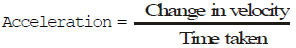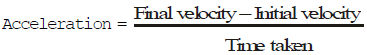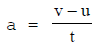v – u = at               v = u + at

Where ; v = final velocity of the body u = initial velocity of the body
a = Acceleration t = time taken

### Second Equation of Motion

It gives the distance travelled by a body in time t.

A body having an initial velocity 'u' acted upon by a uniform acceleration 'a' for time 't' such that final velocity of the body is 'v' and the distance covered is 's'.

The average velocity is given by :-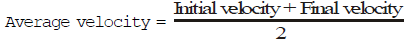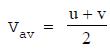distance covered = average velocity × time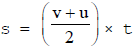but v = u + at (from first equation of motion)

Thus,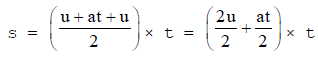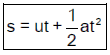Where ; s = distance travelled by the body in time t

u = initial velocity of the body

a = Acceleration

t = time taken

### Third Equation of Motion

A body having an initial velocity 'u' moving with a uniform acceleration 'a' for time 't' such that final velocity 'v' and the distance covered is 's'. the third equation of motion is v2 = u+ 2at. it gives the velocity acquired by a body in travelling a distance s.

v = u + at          .....(i)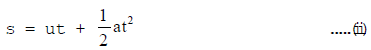Squaring eq. (i), we have

v2 = (u + at)2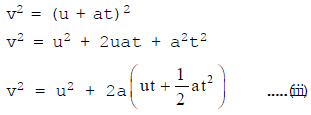Substituting the value of eq (ii) in eq.(iii), we get.  v2 = u2 + 2as

Important Note:

Velocity in m/s = _____ × velocity in km/h.

36 km h-1 = 36000 m h-1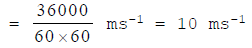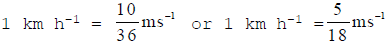To convert km h-1  to ms-1, multiply by 5/18.

To convert ms-1 to km h-1 , multiply by 18/5.

1 km = 1000 m

1m = 100 cm = 1000 mm

Important Points for solving Numericals

• Distance in kilometres should be converted into metre.
• Before solving problems, ensure that the data provided have the same system of unit, i.e. either they should be in SI system or CGS system.
• If a body start from rest, its initial velocity (u) is zero, (u =0)
• If a moving body comes to rest/stops, its final velocity (v) is zero, (v =0)
• If a body is moving with uniform velocity, its acceleration is zero, (a =0)
• If the body is falling under gravity, the value of acceleration should be taken -g.

## Graphical Representation of Motion

### Graph

A graph is a line, straight line or curved, showing the relation between two variable quantities of which one varies as a result of the change in the other.

The quantity which changes independently is called independent variable and the one which changes as a result of the change in the other is called dependent variable.

Plotting a Graph

• Take independent varibale along X-axis and dependent variable along Y-axis.
• Choose convenient scale so that more than 2/3rd of graph is filled.
• Draw free hand curve to join them.

Uses of Graphs

• It gives a bird's eye view of the changes.
• It is used to show dependence of one quantity on the other e.g., distance or velocity on time.
• We can find distance covered in a given time.
• Slope of velocity-time graph gives acceleration.
• We can find position or velocity of body at any instant.
 Competitive WindowA graph gives not only the relation between two variable quantities in a pictorial form but also enable us to study of nature of motion. Speed = slope of distance time graph. If distance-time graph is parallel to time axis, then the body is stationary. If distance-time graph is a straight line having constant gradient or slope, then the body has uniform motion. If distance-time graph is a curve with increasing gradient or slope, then the body has non-uniform motion If slope of distance-time graph increases, the speed of the body increases. If slope of distance-time graph of non-uniform motion decreases, the speed of the body decreases.

### Distance/Displacement-Time Graph

This graph is plotted between the time taken and the distance covered, the time is taken along the x-axis and the distance covered is taken along the y-axis.
Speed = distance/time

• The slope of the distance-time graph gives the speed of the body.
• The slope of the displacement-time graph gives the velocity of the body.

When the body is at Rest

When position of the body does not change with time then it is said to be stationary, the distance-time graph of such a body is a straight line parallel to x-axis.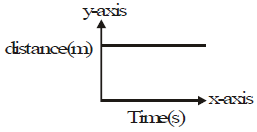Distance-time graph for a Stationary Body

When the body is in Uniform Speed

When the position of the body changes by equal intervals of time then body is said to be moving with uniform speed. The distance-time graph of such a body is a straight line, inclined to x-axis.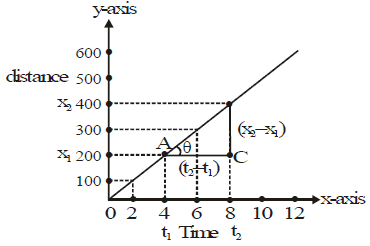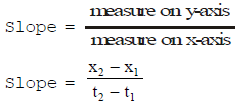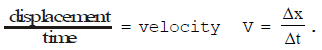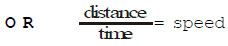Special Case-I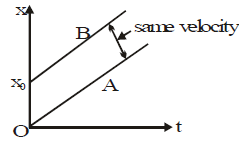In uniform motion along a straight line the position x of the body at any time t is related to the constant velocity as,
xA = vt Starting form zero
xB = x0 + vt starting from x0

Special Case-II

Slope of line A = tanθA = tan0 (∵ θA = 0)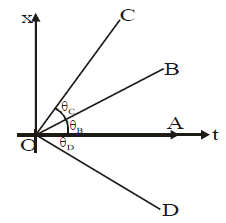=> zero velocity

Slope of line B = tanθB = positive velocity

Slope of line C = tanθc = more positive velocity

∵ θC> θB (tanθC> θ)

Then vC> vB

Slope of line D = tan (-θD) = negative velocity.

When the body is in Motion with a Non-uniform (or Variable) Speed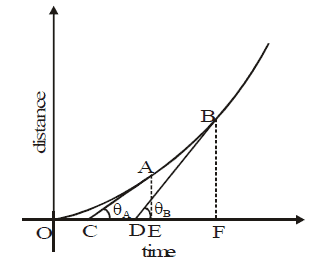Distance-Time graph for a body moving with non-unifrom speed.
The position-time graph is not a straight line, but is a curve.
The speed of the body at any point is known as instantaneous speed and can be calculated by finding the slope at that point.
So instantaneous speed of the body at point A.
Slope at point A = tanθA = AE \ CE
instantaneous speed of the body at point B
Slope at point B = tanθB = BF\DF
θB> θA so slope at point B is greater than the slope at point A.
Hence speed of body at point B is a greater than, the speed of body at point A.

When the Speed Decreases with Passage of Time

Slope at point A > slope at point B (θA> θB)
So, speed at point A > speed at point B

Important Note: A distance time graph can never be parallel to y-axis (representing distance) because this line has slope of 90° and slope = tanθ = tan90° = infinite, which means infinite speed. It is impossible.

Acceleration from Displacement-time Graph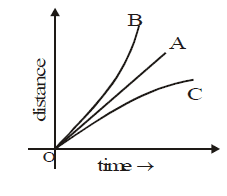For line A : A straight line displacement-time graph represents a

uniform velocity and zero acceleration

for line B : A curved displacement-time graph rising upward represents an increasing velocity and positive acceleration

For line C : A curved displacement-time graph falling downwards, represents a decreasing velocity and negative acceleration.

### Velocity-time Graph

The variation in velocity with time for an object moving in a straight line can be represented by a velocity-time graph. In this graph, time is represented along the x-axis and velocity is represented along the y-axis.
Acceleration = (speed or velocity over time)
hence the slope of the speed/velocity-time graph, gives the acceleration of the body.
Distance = speed × time
hence, area enclosed between the speed-time graph line and x-axis (time axis) gives the distance covered by the body. Similarly area enclosed between the velocity-time graph line and the x-axis (time axis) gives the displacement of the body.

Note: Since the graph takes into account, only the magnitude hence velocity-time graph is not different from speed-time graph.

When the body is moving with Constant Velocity

When the body moves with constant velocity i.e. its motion is uniform.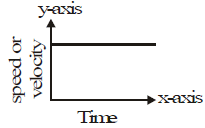The speed or velocity of the body is uniform hence the magnitude remains same. The graph is a straight line parallel to x-axis (time-axis). Since the velocity is uniform. Its acceleration is zero. The slope of the graph in this case is zero.

Conclusion: Velocity-time graph of a body moving with constant velocity is a straight line parallel to time axis.

When the body is moving with Uniform Acceleration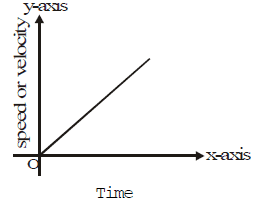The speed or velocity is changing by equal amounts in equal interval of time, the speed or velocity time graph of such a body is a straight line inclined to x-axis (time-axis).

When the body is moving with a Non-uniform (or Variable) Acceleration

The speed or velocity-time graph is not a straight line but is a curve.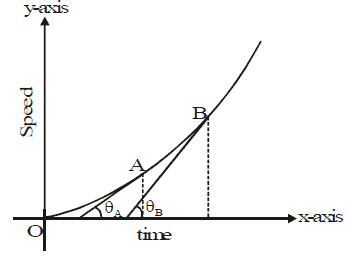The line has different slopes at different times, its acceleration is variable. At point A, slope is less hence acceleratoin is less. At point B slope is more hence acceleration is more.

Note: Speed or velocity-time graph line can never be paralled to y-axis (speed axis), because slope angle becomes 90° than tan90° is infinite it is impossible.

### Distance or Displacement from Speed or Velocity-time Graph.

As distance or displacement = speed or velocity x time, hence the distance or displacement can be calculated from speed or velocity-time graph.

When Speed or Velocity is Uniform (or Constant)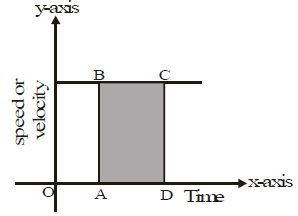Distance/displacement = Area of rectangle ABCD = AB × AD

Thus, We find that the area enclosed by velocity-time graph and the time axis gives the distance travelled by the body.

When Acceleration is Uniform (or Constant)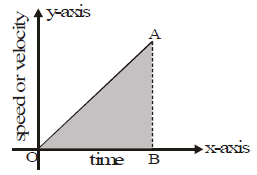distance or displacement = area of right triangle OAB =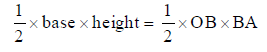When Speed or Velocity as well as Acceleration is Non-uniform (or Variable).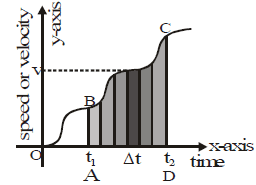The speed-time graph of a body moving irregularly with variable speed and acceleration. For a small interval of time Δt, as there is not much change in speed, hence the speed can be taken as constant.
For this small time interval.
Distance Δs = vΔt = Area of the blackened strip.
For whole time interval between t1 and t2, distance = sum of areas of all the strips, between t1 and t2 = area of shaded figure ABCD.

### Applications of Velocity-time Graphs

A number of useful results can be deduced from velocity time graph.

• Slope of velocity-time graph gives the acceleration.
• Area below velocity-time graph and the time axis gives the distance covered.
• Using the above two results, we can derive all equations of motion.

### Nature of Slopes

Competitive Window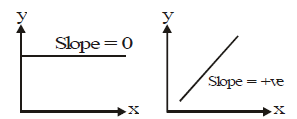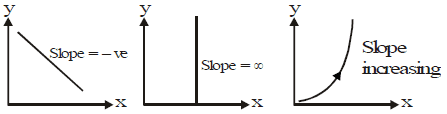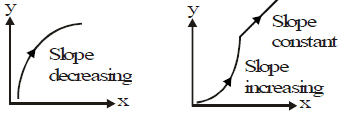## Equations of Motion by Graphical Method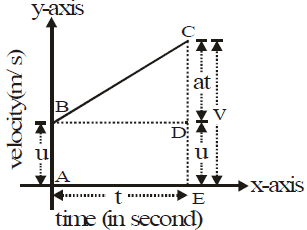Represents a velocity-time graph BC, in which AB represents the initial velocity u, CE represents final velocity v, such that the change in velocity is represented by CD, which takes place in time t, represented by AE.

### Derivation of Velocity-time Relation (v = u + at)

Acceleration = slope of the graph line BC.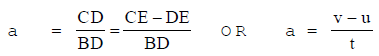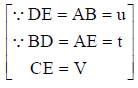v – u = at
v = u + at

### Derivation of Position-time Relation (s = ut + 1/2 at2)

Distance travelled = Area of trapezium ABCE
= Area of rectangle ABDE + Area of triangle BCD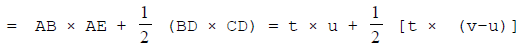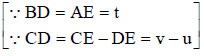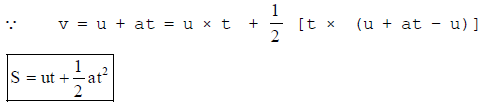### Derivation of Position-Velocity Relation (v2= u2 + 2as)

From the velocity-time graph distance covered = Area of trapezium ABCE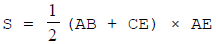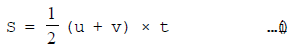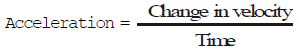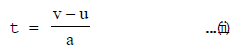Substituting the value of t in eq (i)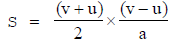∵ A2 – B2 = (A + B) × (A – B)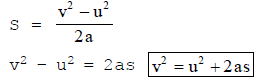The document Graphical Representation of Motion and Equations of Motions by Graphical Method | Physics for Class 9 is a part of the Class 9 Course Physics for Class 9.
All you need of Class 9 at this link: Class 9

## Physics for Class 9

16 videos|69 docs|56 tests

## FAQs on Graphical Representation of Motion and Equations of Motions by Graphical Method - Physics for Class 9

 1. What are the equations of uniformly accelerated motion?Ans. The equations of uniformly accelerated motion are: - v = u + at (final velocity = initial velocity + acceleration x time) - s = ut + 1/2 at^2 (distance traveled = initial velocity x time + 1/2 x acceleration x time^2) - v^2 = u^2 + 2as (final velocity^2 = initial velocity^2 + 2 x acceleration x distance traveled)
 2. What is the graphical representation of motion?Ans. The graphical representation of motion is a graph that shows how an object's position changes with respect to time. The x-axis represents time, while the y-axis represents distance. The slope of the line on the graph represents the object's velocity, and the area under the line represents the object's displacement.
 3. How can we use graphical method to find equations of motion?Ans. We can use the graphical method to find equations of motion by analyzing the motion graphically and finding the slope and area under the line. The slope of the line represents the object's velocity, and we can use it to calculate the acceleration. The area under the line represents the object's displacement, and we can use it to calculate the distance traveled. By combining these values with the initial velocity and time, we can derive the equations of motion.
 4. What is the significance of equations of motion in physics?Ans. The equations of motion are fundamental in physics as they describe how an object moves under the influence of external forces. They are used to calculate the position, velocity, and acceleration of an object at any point in time, and they are essential for understanding how objects behave in the real world. These equations are used in many areas of physics, including mechanics, electromagnetism, and thermodynamics.
 5. What are some real-life applications of uniformly accelerated motion?Ans. Uniformly accelerated motion is a common phenomenon that we observe in our daily lives. Some examples of real-life applications of uniformly accelerated motion include: - A car accelerating from rest to a certain speed on a highway - A ball thrown upwards, accelerating under the force of gravity - A roller coaster gaining speed as it moves down a hill - An elevator accelerating upwards or downwards - A rocket accelerating during launch.

## Physics for Class 9

16 videos|69 docs|56 testsExplore Courses for Class 9 examSignup to see your scores go up within 7 days! Learn & Practice with 1000+ FREE Notes, Videos & Tests.
10M+ students study on EduRev
Track your progress, build streaks, highlight & save important lessons and more!
Related Searches

,

,

,

,

,

,

,

,

,

,

,

,

,

,

,

,

,

,

,

,

,

;